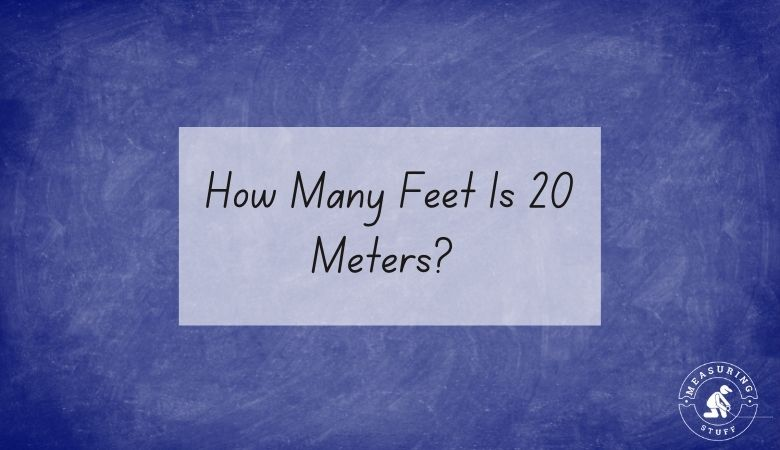# How Many Feet Is 20 Meters? (Easy Conversion Method)

20 meters equals 65.617 feet.

To convert 20 meters into feet, you need to multiply by the conversion factor of 3.281.

For example, use the following formula and multiply the number of meters by 3.281 to get the answer in feet.

20 meters x 3.281 (conversion factor) = 65.617 feet.

This means that there are 65.617 feet in 20 meters.

If you want to reverse the question and figure out how many meters can be made from a certain number of feet, you would divide the number of feet by 3.281.

For example, if you have 20 feet, you can divide it by 3.281 to get 6.096 meters.

This means that there are 6.096 meters in 20 feet.

If you don’t feel like doing the math, use our online conversion calculator below to convert different units of measurements.

## Meters into feet conversion calculator

Use the following calculator to easily convert meters into feet. Simply type in the desired value and select meters in the convert from box and feet in the convert to box. The calculator will instantly do the math for you.

Also, check out all of our online conversion calculators here.

You can also use the following table to convert meters into feet.

## Meters into feet conversion table

You can also convert 20 meters into other units of measurements.

• 20 meters = 787.402 inches
• 20 meters = 2000 centimeters
• 20 meters = 20000 millimeters

Other articles on our site using meters and feet include:

## Feet measurement

A foot is a unit of length measurement that is equal to 12 inches or 0.305 meters.

## Meters measurement

A Meter is part of the metric system of measurement which was created in France to have a way to measure length, mass, and volume. 1 meter is equal to 3 feet 3 inches in length.

## Related articles:

Check out a complete list of related articles in this category here.